# P-divisible group

(diff) ← Older revision | Latest revision (diff) | Newer revision → (diff)

Barsotti–Tate group

A generalization of the concept of a commutative formal group of finite height. The homomorphism induced by multiplication by the prime numberis an epimorphism for a-divisible group.

Letbe a scheme and letbe a prime number. A-divisible group of heightis an inductive system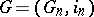of commutative finite group schemesof order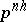(cf. Group scheme) such that the sequences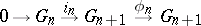are exact (cf. Exact sequence; hereis the homomorphism of multiplication by). A morphism of-divisible groups is a morphism of the inductive systems. A-divisible group is said to be connected (respectively, étale) if allare connected (respectively, étale) group schemes. A connected-divisible group over a field of characteristicis a commutative formal group (considered as the inductive limit of the kernels of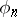, i.e. of multiplication by) for which multiplication byis an isogeny . This fact is generalized to include the case of an arbitrary base schemeon which the homomorphism induced by multiplication byis locally nilpotent . The category of étale-divisible groups is equivalent to the category of-adic representations of the fundamental group of the scheme. Each-divisible groupover an Artinian schemecontains a maximal connected subgroup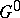, which is known as the connected component of the identity, the quotient by which is an étale-divisible group. The dimension of the Lie algebra of anyis said to be the dimension of the-divisible group.

Letbe an Abelian variety over the fieldof dimension, let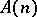be the kernel of the homomorphism of multiplication byinand letbe a natural inclusion. The inductive system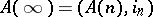is a-divisible group of height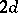. Its connected component of the identity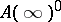coincides with the formal completion ofalong the unit section, while the height ofequals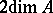.

Letbe a-divisible group of height, let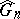denote the Cartier dual finite group schemes and letbe the mapping dual to the mapping of multiplication by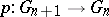. The systemis then a-divisible group of heightand is called the Serre dual to the-divisible group. The sum of the dimensions ofand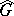is equal to.

As in formal groups, so, too, in-divisible groups, the concept of a Dieudonné module, which plays an important role in the deformation theory of-divisible groups , ,  may be introduced.

Ifis the spectrum of an unequal-characteristic discrete valuation ringwith residue field of characteristic, the structure of a-divisible group is closely connected with the structure of the completion of the algebraic closure of the quotient fieldof, considered as a module over the Galois group of the field.

How to Cite This Entry:
P-divisible group. Encyclopedia of Mathematics. URL: http://encyclopediaofmath.org/index.php?title=P-divisible_group&oldid=13472
This article was adapted from an original article by I.V. Dolgachev (originator), which appeared in Encyclopedia of Mathematics - ISBN 1402006098. See original article